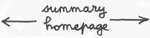2

3 - Third axiom of groups' theory :

Any element of the group must own its inverse , written g-1 , defined by :
(15)

g x g-1 = g-1 x g = 1

In our example :

(16)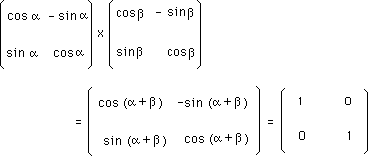i.e : b = - a or :
(17)

g-1 ( a ) = g ( - a )

Here the calculation of the inverse matrix is trivial.

What is the condition for a given square matrix to own its inverse ?

...To any square matrix we can associate a scalar called determinant . For definition see any book devoted to linear calculus . This determinant is codified : det ( g )

In addition we have a general theorem :

det (g1 x g2) = det (g1 ) x det (g1 )

The determinant of a diagonal matrix is :
(18)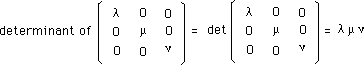As a consequence :

det ( 1 ) = 1

for 1 is a diagonal matrix.

From the definition of the inverse of a matrix :

g x g-1 = g-1 x g = 1

Then :
(19)

det ( g x g-1 ) = det (g) x det (g-1 ) = 1

...If det (g) = 0 the condition (19) cannot be satisfied. Sets of matrixes whose peculiar elements own null determinant don't satisfy the third axiom, and cannot form a group.

By the way :
(20)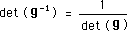4 - Fourth axiom of groups'theory :

The multiplication must be associative, i. e : .
(21)

( g1 x g2 ) x g3 =g1 x ( g2 x g3 )

Matrix multiplication is basically associative.

Dimension of a group :

...As we will see, a group may act on a space whose points are described by column-vectors. For an example space-time points (called "events") :
(22)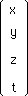...This is a four dimensions space. Different groups may act on it. But the dimension of a group has nothing to do with the dimension of the space it acts on.

The dimension of a group (of matrixes) is the number
of parameters which define these square matrix.

We have given an example of matrixes, defined by a single parameter

a

So that the dimension of this group is one.
Notice that :
(22-bis)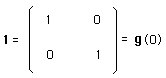Remark :

All groups of matrixes are not commutative , although the group we studied owns this property :
(23)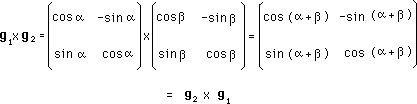If such group acts on a column vector, corresponding to a 2d space :
(23 bis)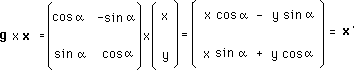it corresponds to rotation around a fixed point, in a plane :

(23 ter)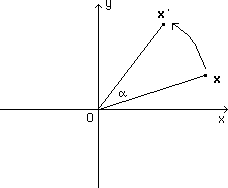This operation is obviously commutative.

You will tend to say : "like all rotations groups".

...You're wrong. Consider the rotations around axis passing by a given point O. Combine two successive rotations, around different axis. This is not commutative. Exercise : show that, using orthogonal axis system (OX, OY, OZ), combined rotations around these axis is not a commutative operation.. Take any object.

- Make a rotation +90° around OX, then a rotation +90° around OZ

- Make a rotation +90° around OZ, then a rotation +90° around OX

Compare the results.

Group's action.

...A group G is composed by square matrixes g . They can be multiplied. We will say that a group may act on itself .
The group may also act on a space, made of points, described by column vectors. Example :

(24)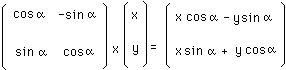If we write :
(25)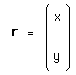the action of the group on this space becomes :
(26)

g x r

...In this peculiar case the action on space identifies to the simple matrix multiplication. But the concept of an action is much more general.